# Introduction

ggplot2.lineplot is an easy to use function to generate line plots in R software using ggplot2 plotting system. It can also be used to customize quickly the plot parameters including main title, axis labels, legend, background and colors. ggplot2.lineplot function is from easyGgplot2 package. An R script is available in the next section to install the package.

At the end of this tutorial you will be able to draw, with few R code, the following plots:ggplot2.lineplot function is described in detail at the end of this document.

# Install and load easyGgplot2 package

easyGgplot2 R package can be installed as follow :

``````install.packages("devtools")
library(devtools)
install_github("easyGgplot2", "kassambara")``````

Load the package using this R code :

``library(easyGgplot2)``

# Data format

The data must be a numeric vector or a data.frame (columns are variables and rows are observations).

`restaurant` data from easyGgplot2 package will be used in the following example.

``````#data.frame
``````##      sex   time total_bill
## 1 Female  Lunch      13.53
## 2 Female Dinner      16.81
## 3   Male  Lunch      16.24
## 4   Male Dinner      17.42``````

# Basic line plot

A subset of `restaurant` data is used :

``````df<-restaurant[c(1,4), c("time", "total_bill")]
``````##     time total_bill
## 1  Lunch      13.53
## 4 Dinner      17.42``````
``````# Basic line plot of the values of "total_bill" variables
ggplot2.lineplot(data=df, xName="time", yName='total_bill')
ggplot2.lineplot(data=df, xName="time", yName='total_bill',
# Use the arrow parameter to add an arrow to the line
# See ?grid::arrow for more details
ggplot2.lineplot(data=df, xName="time", yName='total_bill',
arrow=arrow())
# Add closed arrow to the to end of the line
ggplot2.lineplot(data=df, xName="time", yName='total_bill',
arrow=arrow(angle = 15, ends = "both",
type = "closed")
)``````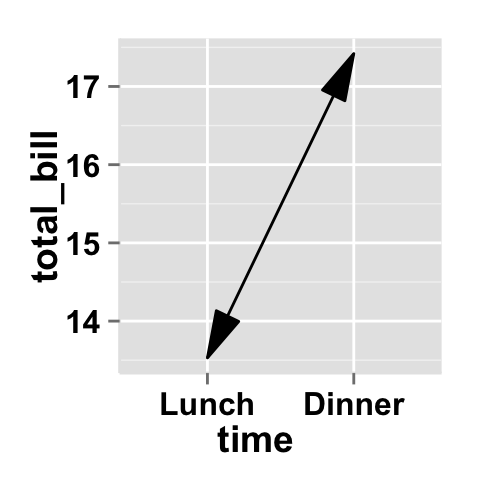# Change the line type and the point shape of line plot

Different point shapes and line types can be used in the plot. By default, ggplot2 uses solid line type and circle shape.

``````# Change color of both line and points
# Change line type and point type,
#and use thicker line and larger points
# Change points to circles with white fill
ggplot2.lineplot(data=df, xName="time", yName='total_bill',
#line color, type and size
color="red", linetype="dotted", size=1.5,
pointFill="white", pointColor="red")``````# Lineplot with more variables

This data will be used for the examples below:

``````df1 <- restaurant
``````##      sex   time total_bill
## 1 Female  Lunch      13.53
## 2 Female Dinner      16.81
## 3   Male  Lunch      16.24
## 4   Male Dinner      17.42``````
``````# Plot of variable 'total_bill' according to xName 'time'.
# The plot is colored by the groupName 'sex'
# line type and point shape are set by the groupName 'sex'
ggplot2.lineplot(data=df1, xName='time', yName="total_bill",
legendPosition="top")
# Use the same linetype and point shape
# but different colors by group
ggplot2.lineplot(data=df1, xName='time', yName="total_bill",
groupName='sex',legendPosition="top",
# Basic plot with black color and
# differents point shapes by group
ggplot2.lineplot(data=df1, xName='time', yName="total_bill",
groupName='sex',legendPosition="top",
# Set point shape manually
ggplot2.lineplot(data=df1, xName='time', yName="total_bill",
groupName='sex',legendPosition="top",
pointSize=3, pointFill="white")+
scale_shape_manual(values=c(22,21))``````## Parameters

The arguments that can be used to customize x and y axis are listed below :

Parameters Description
mainTitle the title of the plot
mainTitleFont a vector of length 3 indicating respectively the size, the style (“italic”, “bold”, “bold.italic”) and the color of x and y axis titles. Default value is: mainTitleFont=c(14, “bold”, “black”).
xShowTitle, yShowTitle if TRUE, x and y axis titles will be shown. Set the value to FALSE to hide axis labels. Default values are `TRUE`.
xtitle, ytitle x and y axis labels. Default values are `NULL`.
xtitleFont, ytitleFont a vector of length 3 indicating respectively the size, the style and the color of x and y axis titles. Possible values for the style:“plain”, “italic”, “bold”, “bold.italic”. Color can be specified as an hexadecimal code (e.g: “#FFCC00”) or by the name (e.g : “red”, “green”). Default values are `xtitleFont=c(14,"bold", "black"), ytitleFont=c(14,"bold", "black")`.
xlim, ylim limit for the x and y axis. Default values are `NULL`.
xScale, yScale x and y axis scales. Possible values : c(“none”, “log2”, “log10”). e.g: yScale=“log2”. Default values are `NULL`.
xShowTickLabel, yShowTickLabel if TRUE, x and y axis tick mark labels will be shown. Default values are `TRUE`.
xTickLabelFont, yTickLabelFont a vector of length 3 indicating respectively the size, the style and the color of x and y axis tick label fonts. Default value are `xTickLabelFont=c(12, "bold", "black"), yTickLabelFont=c(12, "bold", "black")`.
xtickLabelRotation, ytickLabelRotation Rotation angle of x and y axis tick labels. Default value are `0`.
hideAxisTicks if TRUE, x and y axis ticks are hidden. Default value is `FALSE`.
axisLine a vector of length 3 indicating respectively the size, the line type and the color of axis lines. Default value is `c(0.5, "solid", "#E5E5E5")`.

## Main title and axis labels

``````# Change main title and axis titles
ggplot2.lineplot(data=df, xName="time", yName='total_bill',
mainTitle="Total bill\n per time of day",
xtitle="Time of day", ytitle="Total bill")
# Customize title styles. Possible values for the font style :
# 'plain', 'italic', 'bold', 'bold.italic'.
ggplot2.lineplot(data=df, xName="time", yName='total_bill',
mainTitle="Total bill\n per time of day",
xtitle="Time of day", ytitle="Total bill",
mainTitleFont=c(14,"bold.italic", "red"),
xtitleFont=c(14,"bold", "#993333"),
ytitleFont=c(14,"bold", "#993333"))
# Hide x an y axis titles
ggplot2.lineplot(data=df, xName="time", yName='total_bill',
xShowTitle=FALSE, yShowTitle=FALSE)``````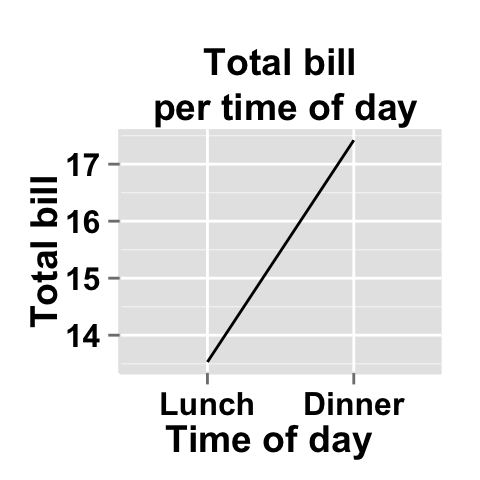## Axis ticks

``````# Axis ticks labels and orientaion
ggplot2.lineplot(data=df, xName="time", yName='total_bill',
xShowTitle=FALSE, yShowTitle=FALSE,
xTickLabelFont=c(14,"bold", "#993333"),
yTickLabelFont=c(14,"bold", "#993333"),
xtickLabelRotation=45, ytickLabelRotation=45)
# Hide axis tick labels
ggplot2.lineplot(data=df, xName="time", yName='total_bill',
xShowTitle=FALSE, yShowTitle=FALSE,
xShowTickLabel=FALSE, yShowTickLabel=FALSE)
# Hide axis ticks
ggplot2.lineplot(data=df, xName="time", yName='total_bill',
xShowTitle=FALSE, yShowTitle=FALSE,
xShowTickLabel=FALSE, yShowTickLabel=FALSE,
hideAxisTicks=TRUE)
# AxisLine : a vector of length 3 indicating the size,
#the line type and the color of axis lines
ggplot2.lineplot(data=df, xName="time", yName='total_bill',
axisLine=c(1, "solid", "darkblue"))``````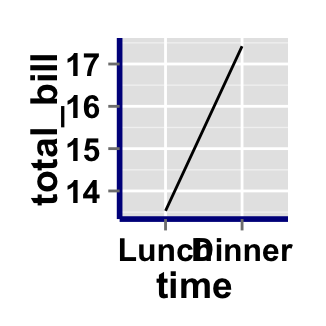## Background and colors

### Change line plot background and fill colors

``````# change background color to "white". Default is "gray"
ggplot2.lineplot(data=df, xName="time", yName='total_bill',
backgroundColor="white")
# Change background color to "lightblue" and grid color to "white"
ggplot2.lineplot(data=df, xName="time", yName='total_bill',
backgroundColor="lightblue", gridColor="white")
# Remove grid; Remove Top and right border around the plot
ggplot2.lineplot(data=df, xName="time", yName='total_bill',
removePanelGrid=TRUE,removePanelBorder=TRUE,
axisLine=c(0.5, "solid", "black"))``````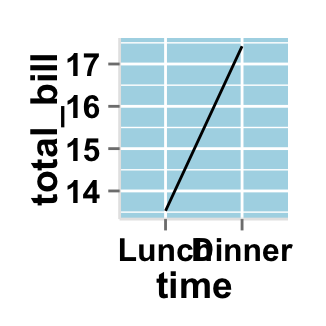### Change line plot color according to the group

Colors can be specified as a hexadecimal RGB triplet, such as `"#FFCC00"` or by names (e.g : `"red"` ). You can also use other color scales, such as ones taken from the RColorBrewer package. The different color systems available in R have been described in detail here.

To change line plot color according to the group, you have to specify the name of the data column containing the groups using the argument `groupName`. Use the argument `groupColors`, to specify colors by `hexadecimal` code or by `name`. In this case, the length of groupColors should be the same as the number of the groups. Use the argument `brewerPalette`, to specify colors using `RColorBrewer`palette.

``````# Color the line plot by groupName
ggplot2.lineplot(data=df1, xName='time', yName="total_bill",
groupName='sex', size=2)
# Change group colors using hexadecimal colors;
ggplot2.lineplot(data=df1, xName='time', yName="total_bill",
groupName='sex',size=2,
backgroundColor="white", #background and line colors
groupColors=c('#999999','#E69F00'))

# Change group colors using brewer palette: "Paired"
ggplot2.lineplot(data=df1, xName='time', yName="total_bill",
groupName='sex',size=2,
backgroundColor="white", #background and line colors
brewerPalette="Paired")
# Change group colors using color names
ggplot2.lineplot(data=df1, xName='time', yName="total_bill",
groupName='sex',size=2,
backgroundColor="white", #background and line colors
groupColors=c('aquamarine3','chartreuse1'))``````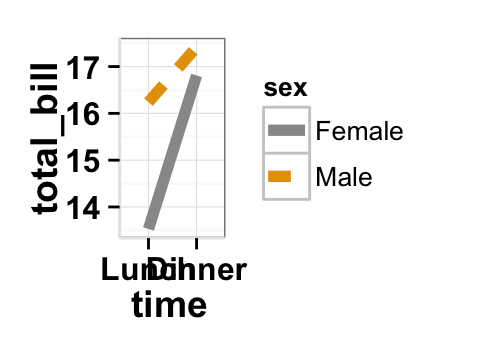## Legend

### Legend position

``````# Change the legend position to "top"
# (possible values: "left","top", "right", "bottom")
ggplot2.lineplot(data=df1, xName='time', yName="total_bill",
groupName='sex',legendPosition="top")

# legendPosition can be also a numeric vector c(x, y)
ggplot2.lineplot(data=df1, xName='time', yName="total_bill",
groupName='sex', legendPosition=c(0.8,0.2))

# Remove plot legend
ggplot2.lineplot(data=df1, xName='time', yName="total_bill",
groupName='sex',showLegend=FALSE)``````It is also possible to position the legend inside the plotting area. You have to indicate the x, y coordinates of legend box. x and y values must be between 0 and 1. c(0,0) corresponds to “bottom left” and c(1,1) corresponds to “top right” position.

### Legend background color, title and text font styles

``````# Change legend background color, title and text font styles
ggplot2.lineplot(data=df1, xName='time', yName="total_bill",
groupName='sex',
#legendPosition=c("right", "left","top", "bottom")
legendPosition="right",
#legendTitleFont=c(size, style, color)
legendTitle="Time", legendTitleFont=c(10, "bold", "blue"),
#legendTextFont=c(size, style, color)
legendTextFont=c(10, "bold.italic", "red"),
#legendBackground: c(fill, lineSize, lineType, lineColor)
legendBackground=c("lightblue", 0.5, "solid", "darkblue" )
)``````## Axis scales

Possible values for y axis scale are “none”, “log2” and log10. Default value is “none”.

``````# Change x axis limit
ggplot2.lineplot(data=df, xName="time", yName='total_bill',
ylim=c(0,18))

# y Log scale. yScale="log2".
# Possible value="none", "log2" and "log10"
ggplot2.lineplot(data=df, xName="time", yName='total_bill',
yScale="log2")``````## Create a customized plots with few R code

``````# Customized lineplot
ggplot2.lineplot(data=df1, xName="time", yName='total_bill',
groupName="sex",size=2,
color="black",
xtitle="Time of day", ytitle="Total bill",
mainTitle="Total bill per time of day")

# Remove grid; Remove Top and right border around the plot;
# different colors per group
ggplot2.lineplot(data=df1, xName="time", yName='total_bill',
groupName="sex",size=2,
groupColors=c('#999999','#E69F00'),
xtitle="Time of day", ytitle="Total bill",
mainTitle="Total bill per time of day",
removePanelGrid=TRUE,removePanelBorder=TRUE,
axisLine=c(0.5, "solid", "black"))``````# Line plot with a numeric x-axis

When the variable on the x-axis is numeric, it is sometimes useful to treat it as continuous, and sometimes useful to treat it as categorical. In this data set, the dose is a numeric variable with values 0.5, 1.0, and 2.0. It might be useful to treat these values as equal categories when making a graph.

This is derived from the ToothGrowth dataset included with R.

``````dfn <- read.table(header=T, text='
supp dose length
OJ  0.5  13.23
OJ  1.0  22.70
OJ  2.0  26.06
VC  0.5   7.98
VC  1.0  16.77
VC  2.0  26.14
')
# line plot with x-axis treated as continuous
ggplot2.lineplot(data=dfn, xName="dose", yName='length',
groupName="supp",
# With x-axis treated as categorical
ggplot2.lineplot(data=dfn, xName="dose", yName='length',
groupName="supp",# ggplot2.lineplot function

## Description

Plot easily a line plot using easyGgplot2 R package.

## usage

``````ggplot2.lineplot(data, xName, yName,  groupName=NULL,
pointFill=NULL, pointColor="black",
arrow=NULL, xAxisType=c("categorical", "continuous"),
groupColors=NULL, brewerPalette=NULL,...)``````

## Arguments

Arguments Descriptions
data data.frame or a numeric vector. Columns are variables and rows are observations.
xName The name of column containing x variable (i.e groups).
yName The name of column containing y variable.
groupName The name of column containing group variable. This variable is used to color plot according to the group.
addPoint If TRUE, points are added to the plot. Default value is FALSE.
pointSize, pointShape, pointFill, pointColor Modify point size, shape, fill and color.
arrow arrow is function from package grid. This function Describe arrows to add to a line. See ?grid::arrow for more details. Usage : arrow=arrow(). Default value is NULL.
xAxisType Indicate the type of x-axis. Possible values =c(“categorical”, “continuous”). Default value is “categorical”. When the variable on the x-axis is numeric, it is sometimes useful to treat it as continuous, and sometimes useful to treat it as categorical.
groupColors Color of groups. groupColors should have the same length as groups.
brewerPalette This can be also used to indicate group colors. In this case the parameter groupColors should be NULL. e.g: brewerPalette=“Paired”.
…. Other arguments passed on to ggplot2.customize custom function or to geom_line functions from ggplot2 package.

The other arguments which can be used are described at this link : ggplot2 customize. They are used to customize the plot (axis, title, background, color, legend, ….) generated using ggplot2 or easyGgplot2 R package.

## Examples

``````library(easyGgplot2)
#data
df<-restaurant[c(1,4), c("time", "total_bill")]
#plot
ggplot2.lineplot(data=df, xName="time", yName='total_bill',
mainTitle="Plot of total bill\n per time of day",
xtitle="Time of day", ytitle="Total bill")
#Or use this
plot<-ggplot2.lineplot(data=df, xName="time", yName='total_bill')
plot<-ggplot2.customize(plot,
mainTitle="Plot of total bill\n per time of day",
xtitle="Time of day", ytitle="Total bill")
print(plot)``````

# Easy ggplot2 ebook

Note that an eBook is available on easyGgplot2 package here.

September 2014 : First edition.

Licence : This document is under creative commons licence (http://creativecommons.org/licenses/by-nc-sa/3.0/).

# Infos

This analysis was performed using R (ver. 3.1.0), easyGgplot2 (ver 1.0.0) and ggplot2 (ver 1.0.0).